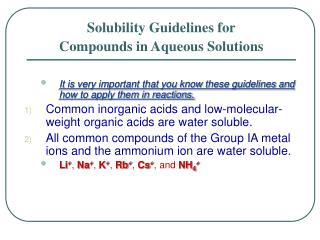Download PresentationIt is very important that you know these guidelines and how to apply them in reactions.

# It is very important that you know these guidelines and how to apply them in reactions. - PowerPoint PPT PresentationDownload Presentation## It is very important that you know these guidelines and how to apply them in reactions.

- - - - - - - - - - - - - - - - - - - - - - - - - - - E N D - - - - - - - - - - - - - - - - - - - - - - - - - - -
##### Presentation Transcript

1. Solubility Guidelines for Compounds in Aqueous Solutions • It is very important that you know these guidelines and how to apply them in reactions. • Common inorganic acids and low-molecular-weight organic acids are water soluble. • All common compounds of the Group IA metal ions and the ammonium ion are water soluble. • Li+, Na+, K+, Rb+, Cs+,and NH4+

2. Aqueous Solutions: An Introduction • Common nitrates, acetates, chlorates, and perchlorates are water soluble. • NO3-, CH3COO-, ClO3-,and ClO4- • Common chlorides are water soluble. • Exceptions – AgCl, Hg2Cl2,& PbCl2 • Common bromides and iodides behave similarly to chlorides. • Common fluorides are water soluble. • Exceptions – MgF2, CaF2, SrF2, BaF2, and PbF2

3. Aqueous Solutions: An Introduction • Common sulfates are water soluble. • Exceptions – PbSO4, BaSO4, & HgSO4 • Moderately soluble – CaSO4, SrSO4,& Ag2SO4 • Common metal hydroxides are water insoluble. • Exceptions – LiOH, NaOH, KOH, RbOH & CsOH • Common bromides and iodides behave similarly to chlorides. • Common fluorides are water soluble. • Exceptions – MgF2, CaF2, SrF2, BaF2, and PbF2

4. Aqueous Solutions: An Introduction • Common carbonates, phosphates, and arsenates are water insoluble. • CO32-, PO43-, & AsO43- • Exceptions- IA metals and NH4+ plus Ca to Ba • Moderately soluble – MgCO3 • Common sulfides are water insoluble. • Exceptions – IA metals and NH4+ plus IIA metals

5. There are three ways to write reactions in aqueous solutions. Molecular equation Show all reactants & products in molecular or ionic form Total ionic equation Show the ions and molecules as they exist in solution Ionic Equations

6. Ionic Equations • Net ionic equation • Shows ions that participate in reaction and removes spectator ions. • Spectator ions do not participate in the reaction. Spectator ions in < >’s.

7. Molecular equation • Total ionic reaction • Net ionic reaction

8. Molecular equation • Total ionic equation • Net ionic equation

9. Molecular equation • Total ionic reaction • Net ionic reaction

10. Molecular equation • Total ionic reaction • Net ionic reaction

11. Oxidation Numbers • Guidelines for assigning oxidation numbers. • The oxidation number of any free, uncombined element is zero. • The oxidation number of an element in a simple (monatomic) ion is the charge on the ion. • In the formula for any compound, the sum of the oxidation numbers of all elements in the compound is zero. • In a polyatomic ion, the sum of the oxidation numbers of the constituent elements is equal to the charge on the ion.

12. Oxidation Numbers • Fluorine has an oxidation number of –1 in its compounds. • Hydrogen, H, has an oxidation number of +1 unless it is combined with metals, where it has the oxidation number -1. • Examples – LiH, BaH2 • Oxygen usually has the oxidation number -2. • Exceptions: • In peroxides O has oxidation number of –1. • Examples - H2O2, CaO2, Na2O2 • In OF2: O has oxidation number of +2.

13. Oxidation Numbers • Use the periodic table to help with assigning oxidation numbers of other elements. • IA metals have oxidation numbers of +1. • IIA metals have oxidation numbers of +2. • IIIA metals have oxidation numbers of +3. • There are a few rare exceptions. • VA elements have oxidation numbers of –3 in binary compounds with H, metals or NH4+. • VIA elements below O have oxidation numbers of –2 in binary compounds with H, metals or NH4+.

14. Assign oxidation numbers to the element specified in each group of compounds • N in NaNO3 • Sn in K2Sn(OH)6 • P in H3PO4 • S in SO32- • Cr in Cr2O72-

15. Assign oxidation numbers to the element specified in each group of compounds • N in NH3 • O in Na2O2 • Fe • N in N2O4

16. Assign oxidation numbers to the element specified in each group of compounds • P in H4P2O7 • Hg in Hg2Cl2 • Li in LiH • S in S4O62- Chemistry is fun!